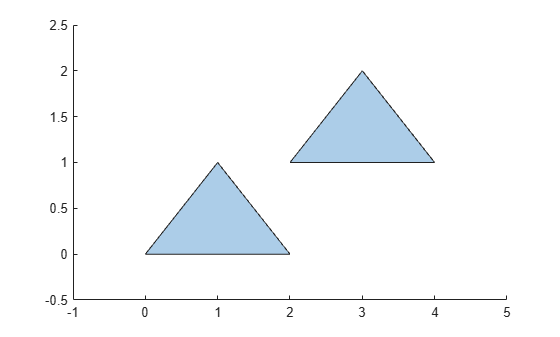# rmboundary

Remove `polyshape` boundary

## Syntax

``polyout = rmboundary(polyin,I)``
``polyout = rmboundary(polyin,I,'Simplify',TF)``

## Description

example

````polyout = rmboundary(polyin,I)` returns a `polyshape` object made up of the polygon `polyin` with the `I`th boundary removed.```
````polyout = rmboundary(polyin,I,'Simplify',TF)` specifies how to treat ill-defined polygons.```

## Examples

collapse all

Create a polygon made up of two triangles, and then remove the triangle whose centroid is nearer to the point (4,2). You can use the `sortboundaries` function to access the boundary index corresponding to the triangle closer to the reference point, which is 1 after sorting.

```x1 = [0 1 2]; y1 = [0 1 0]; x2 = [2 3 4]; y2 = [1 2 1]; polyin = polyshape({x1,x2},{y1,y2}); plot(polyin)``````polysort = sortboundaries(polyin,'centroid','ascend','ReferencePoint',[4 2]); polyout = rmboundary(polysort,1); plot(polyout)```## Input Arguments

collapse all

Input `polyshape`, specified as a scalar.

Data Types: `polyshape`

Boundary index, specified as a scalar integer or vector of integers. Each element of `I` corresponds to a single boundary of the input `polyshape`.

Data Types: `double` | `single` | `int8` | `int16` | `int32` | `int64` | `uint8` | `uint16` | `uint32` | `uint64`

Simplify indicator, specified as one of these values:

• `true` — Alter polygon vertices to produce a well-defined polygon when the input vertices produce intersections or improper nesting.

• `false` — Do not alter input vertices regardless of intersections or improper nesting. Computing with ill-defined polygons can lead to inaccurate or unexpected results.

Data Types: `logical`

## Version History

Introduced in R2017b